JAVA中的数据结构 - 真正的去理解红黑树 原

N阶树-->B（B-）树

1，根和叶子节点都是黑色的

2，不能有有连续两个红色的节点

3， 从任一节点到它所能到达得叶子节点的所有简单路径都包含相同数目的黑色节点

1， 改变颜色

2， 左旋

3， 右旋

//对treeMap的红黑树理解注解. 2017.02.16 by 何锦彬  JDK,1.7.51<br>  <br>/** From CLR */
private void fixAfterInsertion(Entry<K, V> x) {

//新加入红黑树的默认节点就是红色
x.color = RED;
/**
* 1. 如为根节点直接跳出
*/
while (x != null && x != root && x.parent.color == RED) {

if (parentOf(x) == leftOf(parentOf(parentOf(x)))) {

//如果X的父节点（P）是其父节点的父节点（G）的左节点
//即 下面这种情况
/**
*                         G
*               P(RED)              U
*/
//获取其叔(U)节点
Entry<K, V> y = rightOf(parentOf(parentOf(x)));
if (colorOf(y) == RED) {
// 这种情况，对应下面 图：情况一
/**
*                         G
*               P(RED)              U(RED)
*          X
*/
//如果叔节点是红色的（父节点有判断是红色）. 即是双红色，比较好办，通过改变颜色就行. 把P和U都设置成黑色然后,X加到P节点。 G节点当作新加入节点继续迭代
setColor(parentOf(x), BLACK);
setColor(y, BLACK);
setColor(parentOf(parentOf(x)), RED);
x = parentOf(parentOf(x));
} else {
//处理红父，黑叔的情况
if (x == rightOf(parentOf(x))) {
// 这种情况，对应下面 图：情况二
/**
*                           G
*            P(RED)                        U(BLACK)
*                     X
*/
//如果X是右边节点
x = parentOf(x);
// 进行左旋
rotateLeft(x);
}
//左旋后，是这种情况了，对应下面 图：情况三
/**
*                           G
*            P(RED)                        U(BLACK)
*      X
*/

// 到这，X只能是左节点了，而且P是红色，U是黑色的情况
//把P改成黑色，G改成红色，以G为节点进行右旋
setColor(parentOf(x), BLACK);
setColor(parentOf(parentOf(x)), RED);
rotateRight(parentOf(parentOf(x)));
}
} else {
//父节点在右边的
/**
*                         G
*                 U               P(RED)
*/
//获取U
Entry<K, V> y = leftOf(parentOf(parentOf(x)));

if (colorOf(y) == RED) {
//红父红叔的情况
/**
*                          G
*             U(RED)               P(RED)
*/
setColor(parentOf(x), BLACK);
setColor(y, BLACK);
setColor(parentOf(parentOf(x)), RED);
//把G当作新插入的节点继续进行迭代
x = parentOf(parentOf(x));
} else {
//红父黑叔，并且是右父的情况
/**
*                          G
*             U(RED)               P(RED)
*/
if (x == leftOf(parentOf(x))) {
//如果插入的X是左节点
/**
*                            G
*            U(BLACK)                        P(RED)
*                                       X
*/
x = parentOf(x);
//以P为节点进行右旋
rotateRight(x);
}
//右旋后
/**
*                            G
*            U(BLACK)                        P(RED)
*                                                        X
*/
setColor(parentOf(x), BLACK);
setColor(parentOf(parentOf(x)), RED);
//以G为节点进行左旋
rotateLeft(parentOf(parentOf(x)));
}
}
}
//红黑树的根节点始终是黑色
root.color = BLACK;
}

for (int binCount = 0; ; ++binCount) {
if ((e = p.next) == null) {
p.next = newNode(hash, key, value, null);
//JDK8 的hashmap，链表到了8就需要变成颗红黑树了
if (binCount >= TREEIFY_THRESHOLD - 1) // -1 for 1st
treeifyBin(tab, hash);
break;
}

//hashmap的红黑树平衡
static <K,V> TreeNode<K,V> balanceInsertion(TreeNode<K,V> root,
TreeNode<K,V> x) {
x.red = true;
//死循环加变量定义，总感觉JAVA很少这样写代码 哈
for (TreeNode<K,V> xp, xpp, xppl, xppr;;) {
//xp X父节点， XPP X的祖父节点，  XPPL 祖父左节点  XXPR 祖父右节点
if ((xp = x.parent) == null) {
x.red = false;
return x;
}
// 如果父节点是黑色， 或者XP父节点是空，直接返回
else if (!xp.red || (xpp = xp.parent) == null)
return root;

// 下面的代码就和上面的很treeMap像了，

if (xp == (xppl = xpp.left)) {
// 第一种情况, 赋值xppl
//父节点是左节点的情况,下面这种
/**
*                         G
*               P(RED)              U
*/
if ((xppr = xpp.right) != null && xppr.red) {
//如果红叔的情况
// 这种情况，对应下面 图：情况一
/**
*                         G
*               P(RED)              U(RED)
*          X
*/
//改变其颜色，
xppr.red = false;
xp.red = false;
xpp.red = true;
x = xpp;
}
else {
// 黑叔的情况
//  这种情况
/**
*                         G
*               P(RED)              U(BLACK)
*/
if (x == xp.right) {
//如果插入节点在右边 这种
// 这种情况，对应下面 图：情况二
/**
*                           G
*            P(RED)                        U(BLACK)
*                     X
*/
//需要进行左旋
root = rotateLeft(root, x = xp);
xpp = (xp = x.parent) == null ? null : xp.parent;
}
//左旋后情况都是这种了，对应下面 图：情况三
/**
*                           G
*            P(RED)                        U(BLACK)
*      X
*/
// 到这，X只能是左节点了，而且P是红色，U是黑色的情况
if (xp != null) {
//把P改成黑色，G改成红色, 以G为节点进行右旋
xp.red = false;
if (xpp != null) {
xpp.red = true;
root = rotateRight(root, xpp);
}
}
}
}
else {
//父节点在右边的
/**
*                         G
*                 U               P(RED)
*/
//获取U
if (xppl != null && xppl.red) {
//红父红叔的情况
/**
*                           G
*                 U(RED)               P(RED)
*/
xppl.red = false;
xp.red = false;
xpp.red = true;
x = xpp;
}
else {

if (x == xp.left) {
//如果插入的X是右节点
/**
*                            G
*            U(BLACK)                        P(RED)
*                                       X
*/
root = rotateRight(root, x = xp);
xpp = (xp = x.parent) == null ? null : xp.parent;
}
//右旋后
/**
*                            G
*            U(BLACK)                        P(RED)
*                                                        X
*/
if (xp != null) {
//把P改成黑色，G改成红色,
xp.red = false;
if (xpp != null) {
xpp.red = true;
//以G节点左旋
root = rotateLeft(root, xpp);
}
}
}
}
}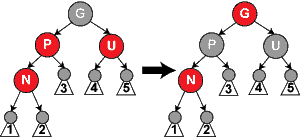情况1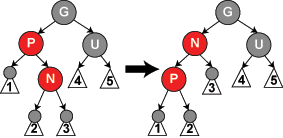情况2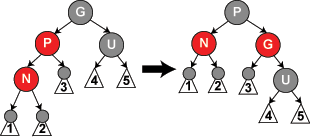情况3

JDK源码处理红黑树的流程图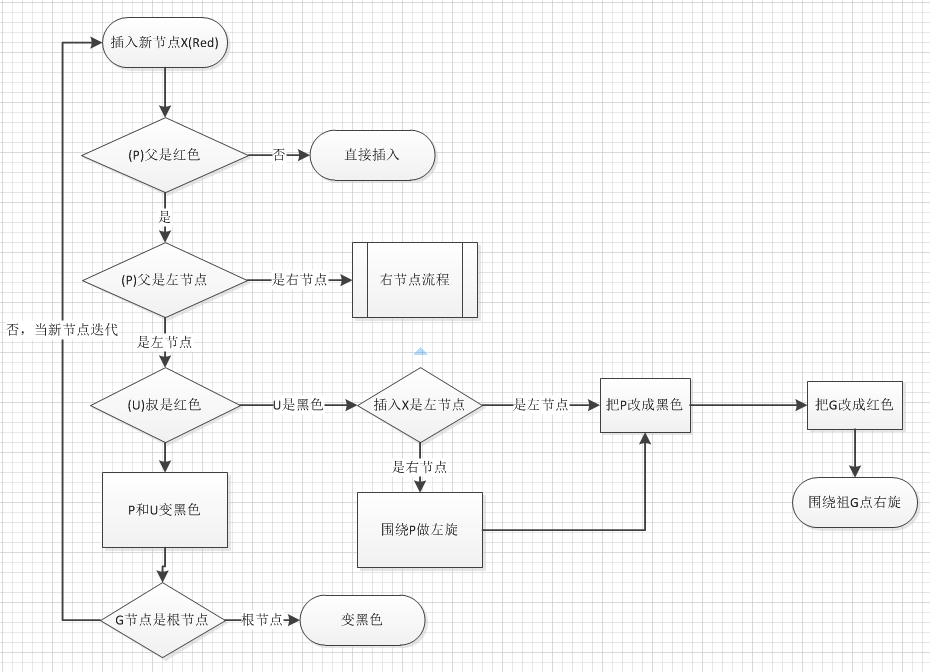1, 如何理解红黑树的O(lgN)的特性？

2, 如何理解红黑树的红黑节点意义？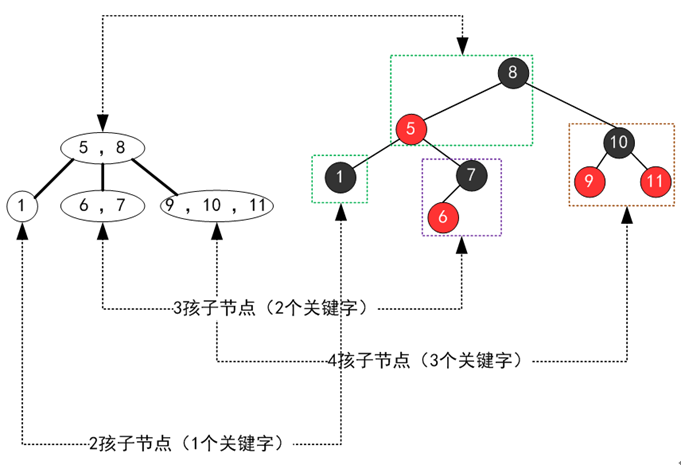（此图转自网上）

3 , B树是如何保持O(lgn)的复杂度的呢?

B+树都是从底布开始往上生长，自动平衡，如 2-3-4树，当节点达到了3个时晋升到上个节点，所以不会产生单独生长一边的情况，形成平衡。

4， 数据库里的索引为什么不用红黑树而是用B+树（Mysql）呢？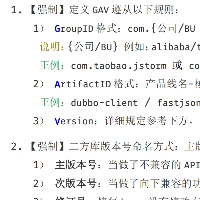爱吃大肉包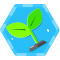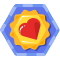《数据结构与算法系列》合集整理

《数据结构与算法系列》合集整理 整理来自博客园skywang12345，以下摘自作者介绍： “最近抽空整理了"数据结构和算法"的相关文章。在整理过程中，对于每种数据结构和算法分别给出"C"、"C++"...

kaixin_code
2018/12/01
185
0

java进阶架构师
2018/08/14
0
0

java进阶架构师
2018/10/28
0
0

内容和标题一样长哦，人家写了好久的。如无特别指明，内容对应的源码是jdk1.7（后面会和1.8对比） 　　1：hashmap简介（如下，数组-链表形式） 　　HashMap的存储结构 　　 　　图中...

java进阶架构师
2018/11/01
0
0

Queue 什么是队列 队列是数据结构中比较重要的一种类型，它支持 FIFO，尾部添加、头部删除（先进队列的元素先出队列），跟我们生活中的排队类似。 队列的种类 单队列（单队列就是常见的队列,...

09/11
22
0

27分钟前
3
0
【2019年8月版本】OCP 071认证考试最新版本的考试原题-第6题

choose three Which three statements are true about indexes and their administration in an Orade database? A) An INVISIBLE index is not maintained when Data Manipulation Language......

oschina_5359
30分钟前
4
0

35分钟前
3
0

Linux磁盘管理好坏直接关系到整个系统的性能问题，Linux磁盘管理常用三个命令为df、du和fdisk。 df df命令参数功能：检查文件系统的磁盘空间占用情况。可以利用该命令来获取硬盘被占用了多少...

xiangyunyan
38分钟前
6
0
js 让textarea的高度自适应父元素的高度

textarea按照普通元素设置height是没有作用的,可以这么来设置, 下面给上一段项目代码 JS代码: \$.fn.extend({ txtaAutoHeight: function () { return this.each(function () {...

39分钟前
5
0